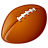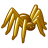# Table to Geodatabase

340
2
06-01-2012 07:13 AMNew Contributor
Hello,

I am trying to import .txt files (tables) into a geodatabase (as a table) using the code below, but am getting the following error:

Traceback (most recent call last):
inTables = inTables + " ; " + tb
TypeError: can only concatenate tuple (not "str") to tuple

Does anyone know what might be causing this and how to fix it?

Thank-you

# Import system modules
import sys, string, os, arcgisscripting

# Create the Geoprocessor object
gp = arcgisscripting.create()

# To import the .txt tables into the geodatabase
# the folder where the original table files are stored
inFolder = "C:\\w\\x\\y\\z\\mnth"
# the geodatabase where the tables will be imported to
outGeodatabase = "C:\\w\\x\\test.gdb"
inTables = ("*", "txt")

gp.Workspace = inFolder

tbs = gp.ListTables()
tb = tbs.Next()
while tb:
if inTables:
inTables = inTables + " ; " + tb
else:
inTables = tb
tb = tbs.Next()

# Put a try-except-look around the tool, in case an error occurs
try:
print "Importing the following tables: " + inTables
gp.TableToGeodatabase(inTables, outGeodatabase)

except:
# If an error occurred, print the messages returned by the tool
print gp.GetMessages()

print "Completed: Table Added to Geodatabase"
Tags (3)
2 RepliesRegular Contributor
You have inTable defined as a tuple (inTables = ("*", "txt")), then you are trying to append a string to it, which is causing the error. Initialize inTables to an empty string, then change the if statement in the while loop to test against the empty string:
```inTables = ""

gp.Workspace = inFolder

tbs = gp.ListTables()
tb = tbs.Next()
while tb:
if inTables == "":
inTables = inTables + " ; " + tb
else:
inTables = tb
tb = tbs.Next()```

Please use CODE tags when submitting code to the forums. It preserves the indenting.Occasional Contributor II
Please use the CODE-Tags ('#'-Button from Editor).

inTables is a Tupel:
`inTables = ("*", "txt")`

Maybe you mean:
`inTables = ""`

So it is an emtpy string.

`if inTables:`

is True, if inTables is not! empty.

Try:
```inTables = ""
tbs = gp.ListTables()
tb = tbs.Next()
while tb:
inTables = inTables + " ; " + tb
tb = tbs.Next()